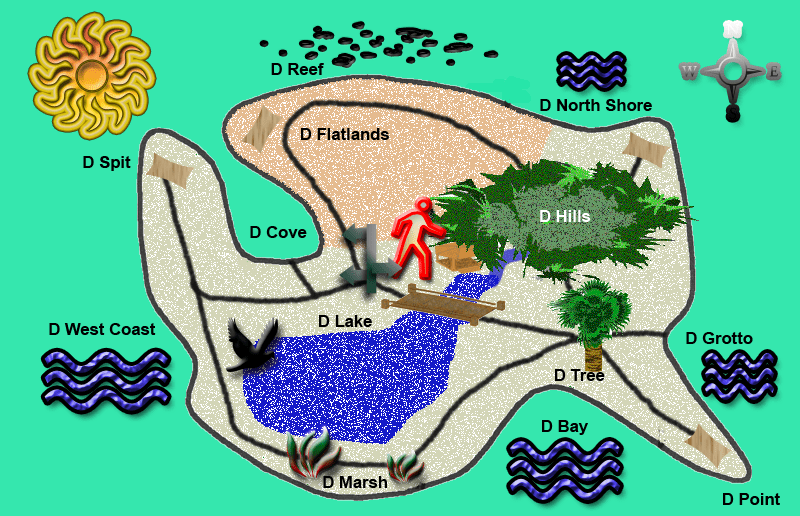# Island Puzzle

In D Land of Dedan there is a saying: “If you can get from Point A to Point B by following a crow, you will be lucky to get there at all”.

The reason for that comes from my own experience when I was exploring D Island following a crow. After traversing throughout and about, I stopped to make a hut so I could rest and store my notes. When I awoke, I was thirsty and went searching for water.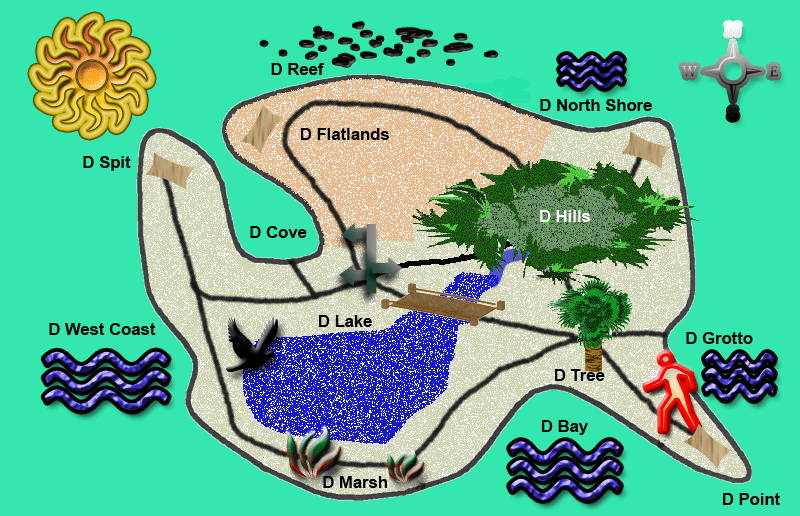Now, all of the paths and the bushes look the same and I can’t find D Hut. I had been following the crow over most of D Island while I was writing my notes just yesterday. As it is a small island, I can make a crude map from guessing the distances between each landmark that I had seen. I also remember that I didn’t go as far as the D West Coast or D Spit and I know it’s between two specific landmarks.

Can you help me find my hut before it gets dark again?

## D Clues

Here is a sketch map, clues and a table.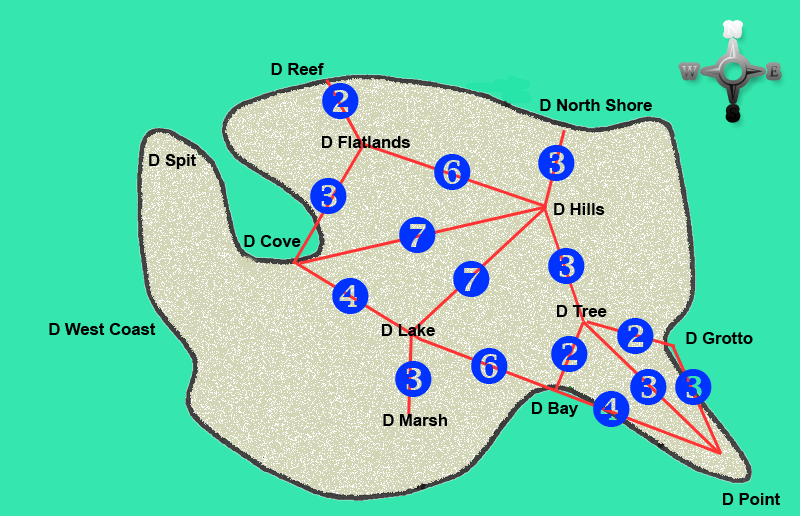Step 1.

Calculate the shortest distance between the all of the landmarks using the map and record the figures into the empty boxes below.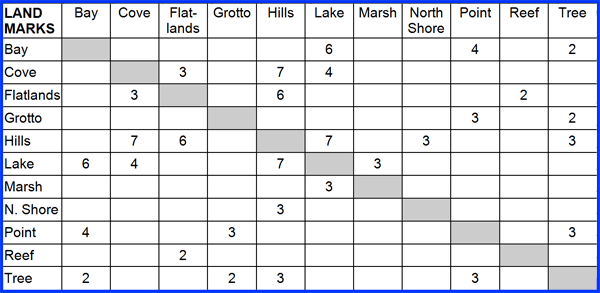Step 2. Along with your table, use the following clues and the process of elimination to find D Hut which is situated between two landmarks. Find the difference between the shortest and the longest distances and divide that number by 2. Cross off all numbers up to and including that number. Count how many times each of the remaining numbers appear in the table. Find the highest amount of times a number appears and divide that number by 2 – and that is how many times our distance number appears in the table. Cross off all other numbers that appear any other number of times. Cross off any number that is the same as the amount of times it appears in the table. You should know the distance number for the location of D Hut by now. D Hut is between one landmark that has one vowel and one landmark that has two vowels. The first letter of these two landmarks are even numbers when counted in the alphabet. The two remaining boxes on your table-graph will have the right answer.

##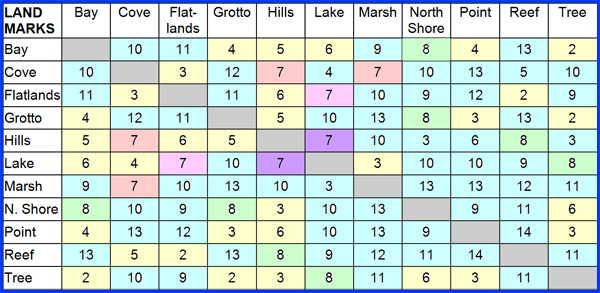1. Yellow. The shortest distance is 2 and the longest distance is 14. The difference is 12, 1/2 of 12 is 6. Cross off all boxes with numbers 2 to 6.
2. Blue. The numbers appear in the table as follows: 7=8; 8=8; 9=10; 10=16; 11=10; 12=6; 13=12; 14=2.  The number that appears the most amount of times 10 at 16 times, 1/2 of 16 is 8, which is the distance number we are looking for. Cross of all boxes with the numbers 9-14.
3. Green. Both 7 and 8 appear in the table 8 times, so the number cannot be 8, it must be 7.
4. Pink. It cannot be between the Lake and the Flatlands as they both have 2 vowels.
5. Orange. It cannot be between the Cove or the Marsh as Cove starts with the 3rd number in the alphabet, while Marsh starts with the 13th letter of the alphabet. Cross off all boxes relating to those areas.
6. Purple. D Hut is between the Lake and the Hills, a distance of 7.